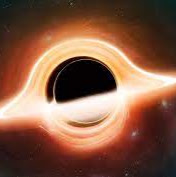### Why is the Moon's orbit so complicated?

•ChristieToWin

one year ago

I like to think I have a good enough understanding of astrophysics but there's still one thing that I just can't explain.

Why does the Moon orbit the Earth the way it does?

Many other moons in the Solar System orbit their planet along the equator and have a nearly circular orbit. After researching, it seems to boil down to two main causes:

• Since every planet rotates on its axis, it has an equatorial bulge, and that extra mass around its equator creates an unequal gravitational field that over millions of years aligns the satellite's orbit around it.

• There's a phenomenon called tidal circularization, which on long timescales, acts to dampen the satellite's eccentricity and make its orbit nearly circular.

Okay, that makes sense for most moons. But our own Moon has an orbit that is notably eccentric and it's also inclined to Earth's equator by an amount that somehow changes over time from 18° to 28°. Why is the Moon's orbit so complicated?I don't have enough confidence or detail to make this an answer, but I think the main things are that the Moon is big compared to the Earth, far from the Earth compared to the size of the Earth and the system as a whole is close to the Sun."Literally every other moon in the Solar System orbits its planet along the equator and has a nearly circular orbit" - Iapetus, Triton, and a whole bunch of outer satellites of the gas giants would disagree...As I've said before, Lunar theory is complicated. ;) Regarding the nodal precession, it helps to think of the Moon's orbit being slightly inclined to the ecliptic and perturbed by the Earth's equatorial bulge. I suppose I should put that in a proper answer... Ah, James beat me to it.Also related: https://astronomy.stackexchange.com/q/10946/16685

•one year ago

The reason the Moon doesn't orbit the Earth's equator is to do with the Laplace plane. This is the plane around which a satellite's orbit precesses: close to the planet, the equatorial bulge is the dominant contribution to the orbital precession, so the plane matches the equatorial plane. Away from the planet, the Sun is the main contribution. The transition between these two regimes occurs around a distance termed the Laplace radius ($$r_\mathrm{L}$$), which is given by:

$$r_\mathrm{L}^5 = J_2' R_\mathrm{p}^2 a_\mathrm{p}^3 \left(1-e_\mathrm{p}^2 \right)^{3/2} \frac{M_\mathrm{p}}{M_\odot}$$

where $$R_\mathrm{p}$$ is the planetary radius, $$a_\mathrm{p}$$ is the planet's semimajor axis, $$e_\mathrm{p}$$ is the planetary orbital eccentricity, $$M_\mathrm{p}$$ is the planet's mass and $$M_\odot$$ is the mass of the Sun.

The quantity $$J_2'$$ is the quadrupole coefficient of the planet and $$n$$ inner satellites (assuming they are in the planet's equatorial plane):

$$J_2' R_\mathrm{p}^2 = J_2 R_\mathrm{p}^2 + \frac{1}{2}\sum_{i=1}^n a_i^2 \frac{m_i}{M_\mathrm{p}}$$

where $$J_2$$ is the planet's quadrupole coefficient and $$a_i$$ and $$m_i$$ are the satellite semimajor axes and masses respectively. For the Earth-Moon system, there are no inner satellites and $$J_2' = J_2$$.

The angle between the planetary spin axis and the Laplace plane $$\phi$$ is given by:

$$\tan 2\phi = \frac{\sin 2\theta}{\cos 2\theta + 2r_\mathrm{L}^5/a^5}$$

where $$\theta$$ is the planetary obliquity. This shows the overall behaviour: for small $$a$$, the denominator tends to infinity and the angle tends to zero. For large $$a$$, the $$2r_\mathrm{L}^5/a^5$$ term vanishes, giving $$\phi = \theta$$. Incidentally this means that the Laplace plane should probably be referred to as a Laplace surface: it isn't actually planar, even though it can be treated as such in the extreme cases.

The above formulae are from the introduction of Nesvorný et al. (2014).

In the case of the Earth, the $$J_2$$ value is 1.08×10-3 (NASA Earth fact sheet), putting the Laplace radius $$r_\mathrm{L}$$ at about 8.4 Earth radii. The Moon's orbit is at 60 Earth radii, which is well beyond the Laplace radius so the Laplace plane is very close to the ecliptic. So even if the orbit of the Moon were in the Earth's equatorial plane, precession would cause it to move out of the plane. An example of a satellite in the transition region is Iapetus (discussed in the Nesvorný et al. paper): note that in the case of Iapetus, there is a significant contribution to $$J_2'$$ from Titan.

The Giant Impact hypothesis for lunar formation predicts that the moon formed close to the Earth in the equatorial plane. The effects of tides caused the Moon to slowly migrate outwards, through the transition region and into its current orbit. The current ~5° inclination of the Moon relative to the Laplace plane is likely a legacy of inclination excitation during the transition. Here's a video of a simulation of the transition by Sarah Stewart-Mukhopadhyay.I really like how you explained everything in detail with the equations +1